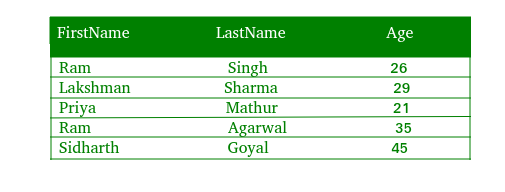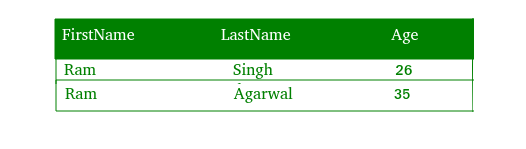# PHP | MySQL WHERE Clause

The WHERE Clause is used to filter only those records that are fulfilled by a specific condition given by the user. in other words, the SQL WHERE clause is used to restrict the number of rows affected by a SELECT, UPDATE or DELETE query.

Syntax :
The basic syntax of the where clause is –
SELECT Column1 , Column2 , ….
FROM Table_Name
WHERE Condition

#### Implementation of WHERE Clause :

Let us consider the following table “Data” with three columns ‘FirstName’, ‘LastName’ and ‘Age’.To select all the rows where the “Firstname” is “ram”, we will use the following code :

Where Clause using Procedural Method :

 ` 0){ ` `        ``echo "<``table``>"; ` `            ``echo "<``tr``>"; ` `                ``echo "<``th``>Firstname"; ` `                ``echo "<``th``>Lastname"; ` `                ``echo "<``th``>age"; ` `            ``echo ""; ` `        ``while(\$row = mysqli_fetch_array(\$res)){ ` `            ``echo "<``tr``>"; ` `                ``echo "<``td``>" . \$row['Firstname'] . ""; ` `                ``echo "<``td``>" . \$row['Lastname'] . ""; ` `                ``echo "<``td``>" . \$row['Age'] . ""; ` `            ``echo ""; ` `        ``} ` `        ``echo ""; ` `        ``mysqli_free_result(\$res); ` `    ``} else{ ` `        ``echo "No Matching records are found."; ` `    ``} ` `} else{ ` `    ``echo "ERROR: Could not able to execute \$sql. "  ` `                                ``. mysqli_error(\$link); ` `} ` ` `  `mysqli_close(\$link); ` `?> `

Output :Code Explanation :

1. The “res” variable stores the data that is returned by the function mysql_query().
2. Everytime mysqli_fetch_array() is invoked, it returns the next row from the res() set.
3. The while loop is used to loop through all the rows of the table “data”.

Where Clause using Object Oriented Method :

 `connect_error); ` `} ` ` `  `\$sql = "SELECT * FROM Data WHERE Firstname='ram'"; ` `if(\$res = \$mysqli->query(\$sql)){ ` `    ``if(\$res->num_rows > 0){ ` `        ``echo "<``table``>"; ` `            ``echo "<``tr``>"; ` `                ``echo "<``th``>Firstname"; ` `                ``echo "<``th``>Lastname"; ` `                ``echo "<``th``>Age"; ` `            ``echo ""; ` `        ``while(\$row = \$res->fetch_array()){ ` `            ``echo "<``tr``>"; ` `                ``echo "<``td``>" . \$row['Firstname'] . ""; ` `                ``echo "<``td``>" . \$row['Lastname'] . ""; ` `                ``echo "<``td``>" . \$row['Age'] . ""; ` `            ``echo ""; ` `        ``} ` `        ``echo ""; ` `        ``\$res->free(); ` `    ``} else{ ` `        ``echo "No matching records are found."; ` `    ``} ` `} else{ ` `    ``echo "ERROR: Could not able to execute \$sql. "  ` `                                    ``. \$mysqli->error; ` `} ` ` `  `\$mysqli->close(); ` `?> `

Output :Where Clause using PDO Method :

 `setAttribute(PDO::ATTR_ERRMODE,  ` `                        ``PDO::ERRMODE_EXCEPTION); ` `} catch(PDOException \$e){ ` `    ``die("ERROR: Could not connect. "  ` `                    ``. \$e->getMessage()); ` `} ` ` `  `try{ ` `    ``\$sql = "SELECT * FROM Data WHERE Firstname='ram'";  ` `    ``\$res = \$pdo->query(\$sql); ` `    ``if(\$res->rowCount() > 0){ ` `        ``echo "<``table``>"; ` `            ``echo "<``tr``>"; ` `                ``echo "<``th``>Firstname"; ` `                ``echo "<``th``>Lastname"; ` `                ``echo "<``th``>Age"; ` `            ``echo ""; ` `        ``while(\$row = \$res->fetch()){ ` `            ``echo "<``tr``>"; ` `                ``echo "<``td``>" . \$row['Firstname'] . ""; ` `                ``echo "<``td``>" . \$row['Lastname'] . ""; ` `                ``echo "<``td``>" . \$row['Age'] . ""; ` `            ``echo ""; ` `        ``} ` `        ``echo ""; ` `        ``unset(\$res); ` `    ``} else{ ` `        ``echo "No records matching are found."; ` `    ``} ` `} catch(PDOException \$e){ ` `    ``die("ERROR: Could not able to execute \$sql. "  ` `                                   ``. \$e->getMessage()); ` `} ` ` `  `unset(\$pdo); ` `?> `

Output :My Personal Notes arrow_drop_upIf you like GeeksforGeeks and would like to contribute, you can also write an article using contribute.geeksforgeeks.org or mail your article to contribute@geeksforgeeks.org. See your article appearing on the GeeksforGeeks main page and help other Geeks.

Please Improve this article if you find anything incorrect by clicking on the "Improve Article" button below.

Article Tags :
Practice Tags :

Be the First to upvote.

Please write to us at contribute@geeksforgeeks.org to report any issue with the above content.